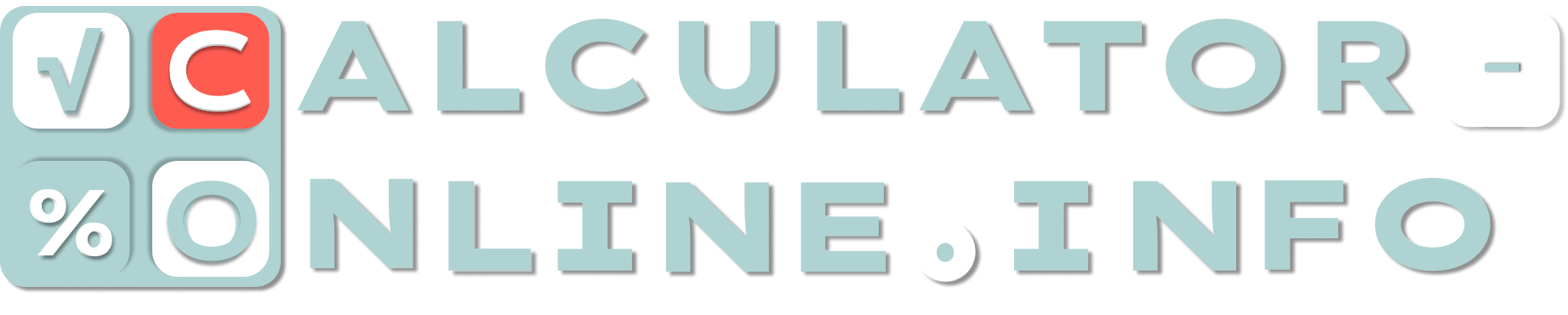# Percent Calculator

Calculate the percentage
%
of
=
0.00
×
Number
is what percent of
=
0.00 %
×
%
to
=
0.00
×
Subtract this percentage
%
from
=
0.00
×
Of what number
is
%
=
0.00
×
What is the percent change from
to
=
0.00 %
×
Number of digits after the decimal point

If you need to calculate percentage of a number, you can do any math operation here. There are 6 helpful calculators that are used for different purposes:

## Calculate percentage of a number

• The first percentage calculator is for classic operations. It answers the question “How to find percentage of number?”. For example: what is 15% of 500?

## What percent of a number is another number?

• The second percentage calculator is the tool that calculates percentage between two numbers. For example: 45 is what percent of 300.

• The third one is necessary to add percent to number. It might be used as surcharge calculator, tips calculator, tax calculator or some else when you need to add a percentage. For example: \$1500 + 12% or 5000 + 36.5%.

## Subtract percentage calculator

• The next one is subtract percent calculator. Similar to previous calculator it can subtract percentage. Such a tool makes it possible to calculate discounted price as well as any other value. For example: \$500 – 25% or 30 586 – 11,4%.

## Reverse percentage calculator

• The fifth percent calculator is the example of a reverse percentage calculator when you need to check a number as a percent of another number. For example: 55 is 25% of what number.

## Increase & decrease percentage calculator

• The last calculator is decrease/increase percentage calculator which is needed for comparison of two numbers. This tool can calculate percent difference and show percent change between to numbers. For example: what is percent change from 500 to 350 or what is the percentage decrease from 135 to 25?

## How to calculate percentage?

Depending on what exactly you need to calculate, you should enter only two parameters: a number and a percentage or two numbers. If you want to delete the data you’ve entered, click on the cross button.

Rating
( 13 assessment, average 5 from 5 )